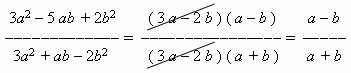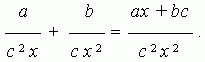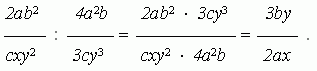# Algebraic fractions

Algebraic fraction. Canceling fractions.
Multiplication and division of fractions.

Algebraic fraction is an expression of a shape A / B , where A and B can be a number, a monomial, a polynomial. As in arithmetic, A is called a numerator , B – a denominator . Arithmetical fraction is a particular case of an algebraic one.

Canceling fractions

E x a m p l e :